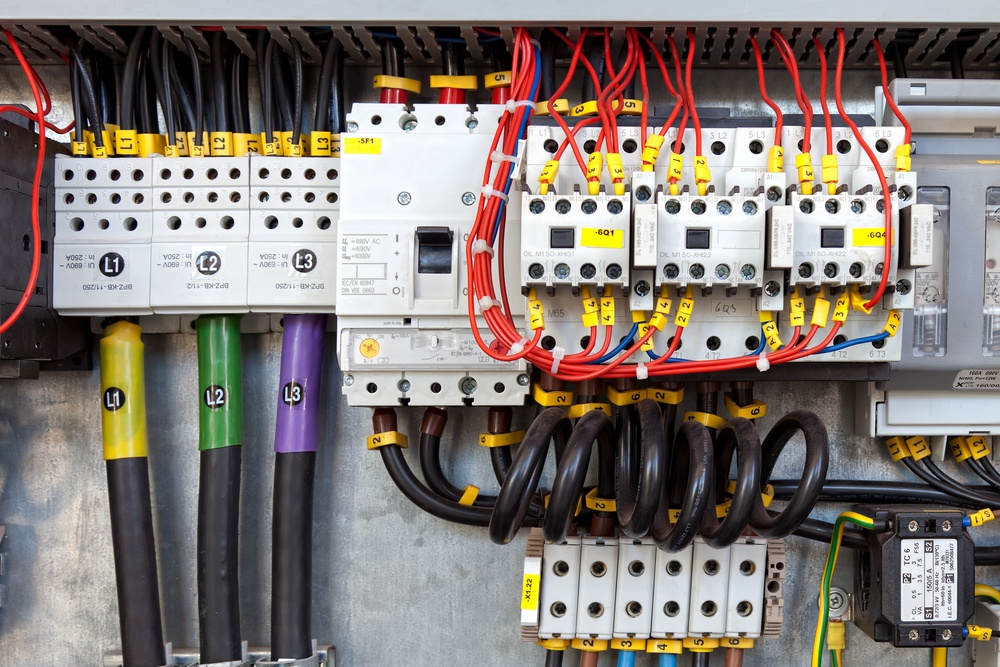When constructing a building in Chicago, you may want to find out the maximum electric power that the building can have and also assess the distribution systems. This helps in efficient planning and budgeting of electrical connections during the construction project. For instance, you will know how many transformers to install to supply the estimated maximum electrical load.

The best way to achieve this mission is to estimate the electrical load for the building at the early stage of the project, and this can be achieved easily with the help of a certified MEP engineering services. This article introduces the concept and further discusses the important load estimating factors to consider.

Get electrical load estimates of your Chicago buildings from our certified electrical design engineers.Importance of Electrical Load Estimation in Chicago Buildings

The importance of electrical load estimation need not be overlooked in Chicago buildings as it carries many benefits. Normally, the estimations are done in the draft design of the building to plan electrical installation as a result of the electric capacity.

The electrical design engineer can help plan the circuit configurations and connections throughout the building. As a building owner, you will know how much to budget for the required number of transformers, main switchgear room and other substations if need be. Also, when applying to the power supply company, you will have clear requirements of your electricity needs as per the estimated loads.

Electrical load estimation requires an in-depth analysis of electrical load characteristics. It takes into consideration the demand factor relationship between the total connected loads and the actual demand imposed on the electric system. Let’s first explain the important load estimating factors below: demand factor, coincidence factor, load factor, maximum demand and diversity factor.

Demand factor – the demand factor is defined as the ratio of the maximum demand over the total connected loads of the system; which is expressed mathematically as:

Demand factor =maximum demand load/total connected loads

Coincidence factor – the coincidence factor is defined at the ratio of the maximum demand or a portion of it over the total of the individual maximum demands of the subdivisions; expressed mathematically as maximum demand of a system/total of individual maximum demands. In actual sense, the electrical loads seldom occur simultaneously but there is some degree of diversity hence the calculation of the coincidence factor, also referred to as the factor of simultaneity (ks).

Diversity factor – diversity factor is regarded as the reciprocal of the coincidence factor; that is, it is the ratio of the total of individual demands over the maximum demand of a system. Note the main difference between the demand and diversity factor. The demand factor, for instance, applies to individual loads whereas the diversity factor applies on load groups.

Load factor – it is the average load over a certain period of time over the maximum load in that designated period. Load factor = average load/maximum load

Maximum demand – is the total demand of the system for specific intervals

Methods of Estimating Electrical Load in Chicago Buildings

The calculation of load estimates may seem too technical for homeowners in Chicago. However, a certified electrical design engineer will be able to complete the process easily. For expedited landlord approvals, construction cost bidding and an efficient construction process, building designs have to be signed by a certified electrical engineer.

Wrap up

Electrical load refers to the total electric power consumed by all electrical and electronic appliances in the building. An estimation of this load helps homeowners to estimate the budget needed for maximum power demands and distribution systems. This makes construction process seamless. The aforementioned terminologies are taken into account when estimating the electrical load.

New York Engineers have a team of experienced and expert electrical engineers to provide electrical engineering services for Chicago buildings. The engineers can prepare electrical designs that include electrical riser diagrams, lighting, mechanical power, and panel board schedules from low to high voltage systems based on electrical load estimations.

### Related Articles#### Chicago Department of Buildings Electrical Power Connection Requirements for Sit/Stand Motorized Desks